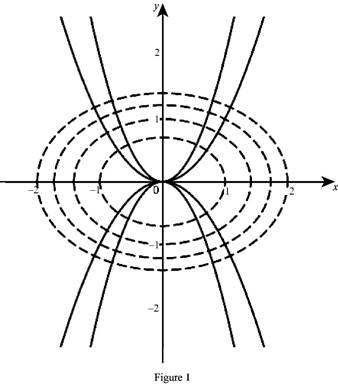# One family of the curves are orthogonal trajectories to the other family.### Single Variable Calculus: Concepts...

4th Edition
James Stewart
Publisher: Cengage Learning
ISBN: 9781337687805### Single Variable Calculus: Concepts...

4th Edition
James Stewart
Publisher: Cengage Learning
ISBN: 9781337687805

#### Solutions

Chapter 3.5, Problem 43E
To determine

## To show: One family of the curves are orthogonal trajectories to the other family.

Expert Solution

### Explanation of Solution

Given:

The curves y=cx2 and x2+2y2=k.

Derivative rules:

Chain rule: dydx=dydududx

Proof:

Obtain the slope of the curves y=cx2.

ddx(y)=ddx(cx2)dydx=cddx(x2)=2cx

Thus the slope of the tangent to the curve y=cx2 is m1=2cx.

Obtain the slope of the curves x2+y2=k.

ddx(x2+y2)=ddx(k)ddx(x2)+2ddx(y2)=02x+4ydydx=0dydx=x2y

Thus, the slope of the equation x2+y2=k is m2=x2y.

The product slope is computed as follows,

m1m2=2cx(x2y)=2cx22y=2y2y       [Qy=cx2]=1

If c0 then the family of the curves orthogonal trajectories to another family of the curves.

If c=0, then

y=(0)x2=0

Substitute the value y=0 in the equation x2+y2=k.

x2+(0)2=kx2=kx=±k

The line y=0 is horizontal line and it intersect x2+2y2=k at the points (k,0) and (k,0). That is, the curve x2+2y2=k has vertical tangent at the points (k,0) and (k,0).

Therefore, the curves y=cx2 and x2+y2=k are orthogonal trajectories to each other is proved.

Graph:

The family of the curves y=cx2 and x2+y2=k as shown below in Figure 1.From the graph, it is observed that the family of the curves y=cx2 and x2+y2=k are orthogonal trajectories to each other.

### Have a homework question?

Subscribe to bartleby learn! Ask subject matter experts 30 homework questions each month. Plus, you’ll have access to millions of step-by-step textbook answers!